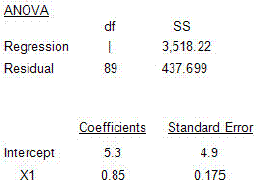### CFA Practice Question

There are 208 practice questions for this study session.

### CFA Practice Question

In comparing the relation of percentage change in company sales with GDP growth, your regression software provides the following output:Assuming that GDP next year is expected to decline by 3.2%, what is your best estimate for next years percentage change in sales?
A. 2.58%
B. 4.96%
C. 8.02%
Explanation: %ΔSales = 5.3 + 0.85 (%ΔGDP) = 5.3 + 0.85 (-3.2%) = 2.58%.